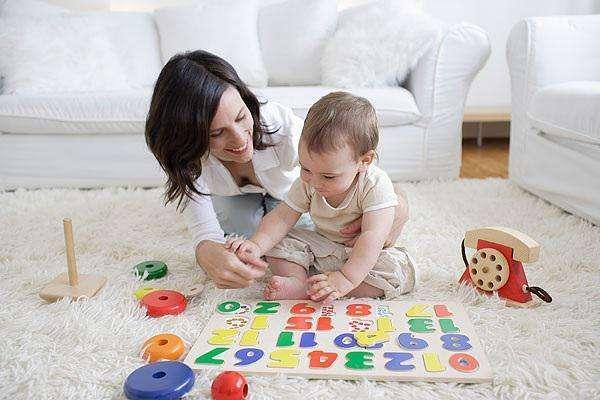# 早教中心教案：蒙特梭利启蒙课程教案 跑跑班

× ×  ×/ × ×  ×/ 欢迎 你和/ × ×× ×  ×/ × ×  ×/ 欢迎 你和/ × ×× ×  ×/ × ×  ×/ 欢迎 你和/ × ×× ×  ×/ × ×  ×/ 欢迎 你和/ × ×

× ×  ×/ × ×  ×/ 欢迎 你和/ × ×

5 3  6 6 | 5 - | 3 5  5 1 | 2 - | 5 3  6 6 | 5 - | 3 5  5 1 | 2 - |

6  6 | 6 5  3 | 6  6 | 6 5  3 | 5  5 | 1 2  3 | 2 2  3 5 | 1- ||

× ×  ×/ × ×  ×/ 欢迎 你和/ × ×

× ×  ×/ × ×  ×/ 欢迎 你和/ × ×

1 1  2 3 | 1 5 | 1 1  2 3 | 1 5 |

.  .

1 1  2 3 | 4 3  2 1 | 7 5  6 7 | 1 1 1 ||

. . . .

× ×  ×/ × ×  ×/ 欢迎 你和/ × ×

1 5  5 1 5  5 | 1 2 3 2 5 - | 5 6 5 4 3 4 3 2 | 1 2 3 - |

. .   . .

1 5  5 1 5  5 | 1 2 3 2 5 - | 5 6 5 4 3 4 3 2 | 6 7 1 - ||

. .   . .

× ×  ×/ × ×  ×/ 欢迎 你和/ × ×

× ×  ×/ × ×  ×/ 欢迎 你和/ × ×

× ×  ×/ × ×  ×/ 欢迎 你和/ × ×

× ×  ×/ × ×  ×/ 欢迎 你和/ × ×